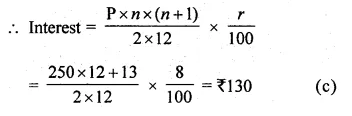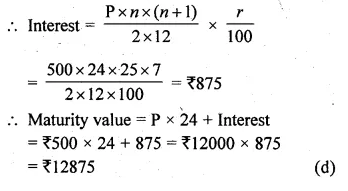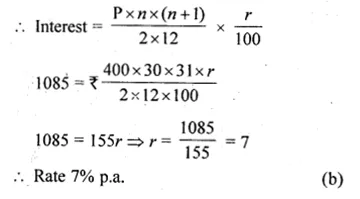## ML Aggarwal Class 10 Solutions for ICSE Maths Chapter 2 Banking MCQS

These Solutions are part of ML Aggarwal Class 10 Solutions for ICSE Maths. Here we have given ML Aggarwal Class 10 Solutions for ICSE Maths Chapter 2 Banking MCQS

More Exercises

Choose the correct answer from the given four options (1 to 4) :

Question 1.
If Sharukh opened a recurring deposit account in a bank and deposited Rs 800 per month for $$1 \frac { 1 }{ 2 }$$ years, then the total money
deposited in the account is
(a) Rs 11400
(b) Rs 14400
(c) Rs 13680
(d) none of these
Solution:
Monthly deposit = Rs800
Period (n) = $$1 \frac { 1 }{ 2 }$$ years = 18 months
.’. Total money deposit = Rs 800 x 18
= Rs 14400 (b)

Question 2.
Mrs. Asha Mehta deposit Rs 250 per month for one year in a bank’s recurring deposit account. If the rate of (simple) interest is 8% per annum, then the interest earned by her on this account is
(a) Rs 65
(b) Rs 120
(c) Rs 130
(d) Rs 260
Solution:
Deposit per month (P) = Rs 250
Period (n) = 1 year = 12 months
Rate (r) = 8% p.a.Question 3.
Mr. Sharma deposited Rs 500 every month in a cumulative deposit account for 2 years. If the bank pays interest at the rate of 7% per annum, then the amount he gets on maturity is
(a) Rs 875
(b) Rs 6875
(c) Rs 10875
(d) Rs 12875
Solution:
Deposit (P) = Rs 500 per month
Period (n) = 2 years = 24 months
Rate (r) = 7% p.a.Question 4.
John deposited Rs 400 every month in a bank’s recurring deposit account for $$2 \frac { 1 }{ 2 }$$ years. If he gets Rs 1085 as interest at the time of maturity, then the rate of interest per annum is
(a) 6%
(b) 7%
(c) 8%
(d) 9%
Solution:
Deposit (P) = Rs 400 per month
Period (n) = $$2 \frac { 1 }{ 2 }$$ years = 3 months
Interest = Rs 1085
Let r% be the rate of interestHope given ML Aggarwal Class 10 Solutions for ICSE Maths Chapter 2 Banking MCQS are helpful to complete your math homework.

If you have any doubts, please comment below. Learn Insta try to provide online math tutoring for you.